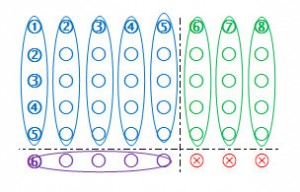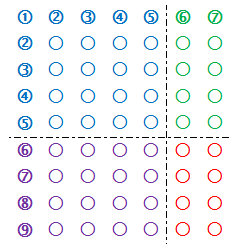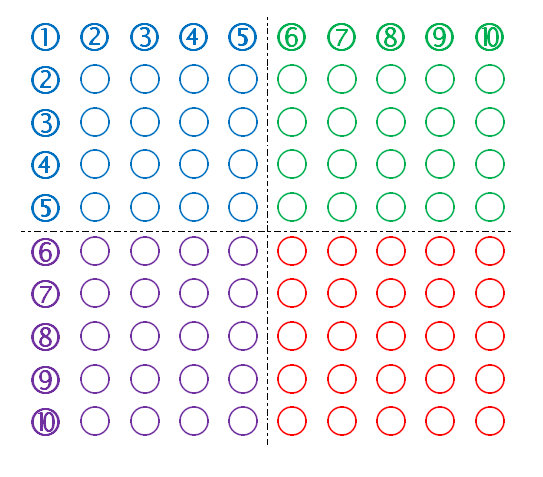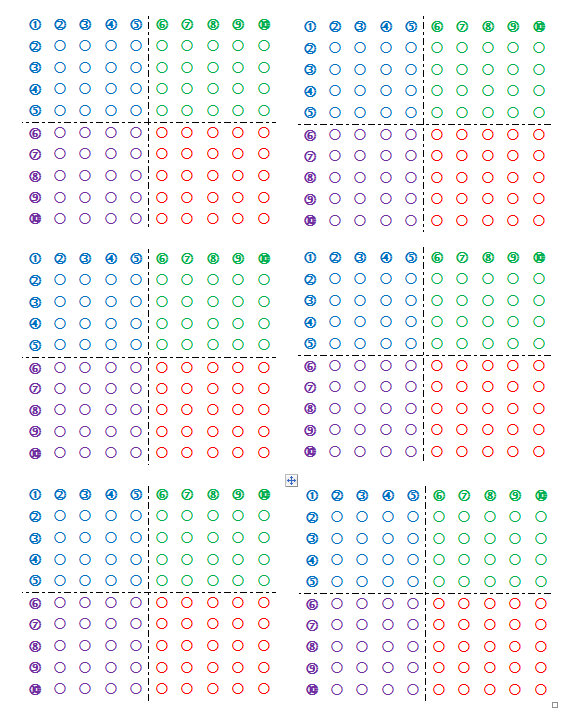# Multiplication foldable arrays to learn multiplication facts greater than five

The fold-able array below has 10 x 10 = 100 dots.  Each of the four colored parts is 5 x 5 =25.

The product of two numbers is shown as groups of 5 plus some extra.  Use the five times multiplication table and add the extra’s. It will help children when you fold the numbers that are not used to the back.

Example 1:

What is 6 x 8? It is 6 rows of 8. Fold back between the 8 and 9 column, so you only see rows of 8. Fold between the 6 and 7 row, so you only see 6 rows.

Count the total: 5 blue groups of five, 3 green groups of five, 1 purple group of five:

5 + 3 + 1 = 9 groups of five. Nine groups of five = 45. Now add the extra red dots: 45 + 3 = 48.Some children see immediately that the blue section is 25 + 3 green fives and one purple 5 = 45 and start to count the extra 3 red dots:

45 + 3 = 48.

Some children like to color in or connect the groups of five dots with a line to make it easier to count the groups of five.

Example 2:

What is 9 x 7? It is 9 rows of 7. Fold back between the 7 and 8 column, so you only see rows of 7. Fold between the 9 and 10 row, so you see 9 rows.

Count the total: 5 blue groups of five, 2 green groups of five, 4 purple groups of five

5 + 2 + 4 = 11 groups of five.Eleven groups of five = 55.

Now add the extra red dots:

55 + 8 = 63.

Note: this method links multiplication to area and lays the foundation to later grasp the distributive property and FOIL method to multiply polynomials: (5+4)(5+2) = 25+10+20+8